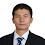### [How To] 在Blogger上使用MathJax

這篇基本上算是紀錄我如何在Blogger成功安裝MathJax的筆記。其實從很早以前，我就有著要把自己所學到的流病與統計知識一點一滴的紀錄上來，因此曾經裝過一次，但我卻想不起來該怎麼使用他，所以只好一切從頭。不過也在這個重新摸索的過程中，發現到許多MathJax成長的地方，也算是另一種收穫吧？

#### 範例與原始碼

##### 範例

MathJax有兩種不同的用法，一種是displayMath，另一種則是inlineMath

displayMath：使用"${$$}"以及"{$$}$"包夾TEX語法

$$\left( \sum_{k=1}^n a_k b_k \right)^2 \leq \left( \sum_{k=1}^n a_k^2 \right) \left( \sum_{k=1}^n b_k^2 \right)$$
$$\mathbf{V}_1 \times \mathbf{V}_2 = \begin{vmatrix} \mathbf{i} & \mathbf{j} & \mathbf{k} \\ \frac{\partial X}{\partial u} & \frac{\partial Y}{\partial u} & 0 \\ \frac{\partial X}{\partial v} & \frac{\partial Y}{\partial v} & 0 \end{vmatrix}$$

inlineMath：使用"${$}$"以及"${$}$"包夾TEX語法

#### 延伸閱讀

http://docs.mathjax.org/en/latest/start.html#mathjax-cdn

### 留言Unknown寫道…Yubao寫道…

1. 这个我试了，不显示

$$\mathbf{V}_1 \times \mathbf{V}_2 = \begin{vmatrix} \mathbf{i} & \mathbf{j} & \mathbf{k} \\ \frac{\partial X}{\partial u} & \frac{\partial Y}{\partial u} & 0 \\ \frac{\partial X}{\partial v} & \frac{\partial Y}{\partial v} & 0 \end{vmatrix}$$

2. 多行如何显示，例如：

$$a^2+b=c$$